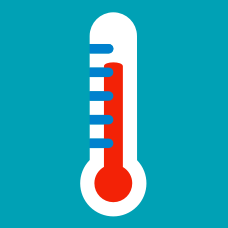Classical Mechanics

# Ideal gas law

Consider an oxygen gas with a volume of $1000 \text{ cm}^3$ at $45.0^\circ\text{C}$ and $1.03 \times 10^5 \text{ Pa}.$ If it expands until its volume is $1600 \text{ cm}^3$ and its pressure is $1.06 \times 10^5 \text{ Pa},$ what is the approximate final temperature of the gas?

The value of the gas constant is $R=8.31 \text{ J/mol}\cdot\text{K}.$

When the temperature is $0.00^\circ\text{C},$ a tire has a volume of $1.63 \times 10^{-2} \text{ m}^3$ and a gauge pressure of $170 \text{ kPa}.$ Assuming that the atmospheric pressure is $1.01 \times 10^5 \text{ Pa},$ what is the approximate gauge pressure of the air in the tires when its temperature rises to $27.0^\circ\text{C}$ and its volume increases to $1.68 \times 10^{-2} \text{ m}^3?$

Suppose a cylinder containing $8 \text{ L}$ of oxygen gas. The temperature and pressure of it is $20^\circ\text{C}$ and $12 \text{ atm},$ respectively. If the temperature is raised to $36 ^\circ\text{C},$ and the volume is reduced to $6.5 \text{ L},$ what will be the approximate final pressure of the gas in atmospheres?

Assume that the gas is ideal.

If one mole of ideal gas expands at a constant temperature $T$ of $320 \text{ K}$ from an initial volume $V_i$ of $15 \text{ L}$ to a final volume $V_f$ of $19 \text{ L},$ Approximately how much work is done by the gas during the expansion?

The value of the gas constant is $R=8.31 \text{ J/mol}\cdot\text{K}.$

Suppose that $1.25 \times 10^{14}$ particles traveling in the positive $x$-direction in a vacuum chamber at a speed of $3.00 \times 10^7 \text{ m/s}$ strike a circular target of radius $4.00 \text{ mm}$ during $4.00 \times 10^{-8} \text{ s}.$ The mass of each particle is $9.11 \times 10^{-31} \text{ kg}.$ What is the average pressure felt by the target, assuming that all the particles penetrate the target and are absorbed?

×